# Okasaki's PFDS, Chapter 3

This post contains my solutions to the exercises in chapter 3 of Okasaki’s ‘Purely Functional Data Structures’. The latest source code can be found in my GitHub repo.

## Leftist Trees

The right spine of a binary tree is the rightmost path from that node to an empty node. For example, the empty tree has a right spine of length 0.

A binary tree is said to satisfy the leftist property if every node has the property that the rank of its left child (= length of its right spine) is greater than or equal to the rank of its right child. A leftist tree is a binary tree with the leftist property.

The following are some examples of (the shape of) leftist trees where the keys have been omitted. The number at each node instead indicates the length of its right spine and any blank nodes are empty nodes.The only leftist tree with 1 node.The only leftist tree with 2 nodes.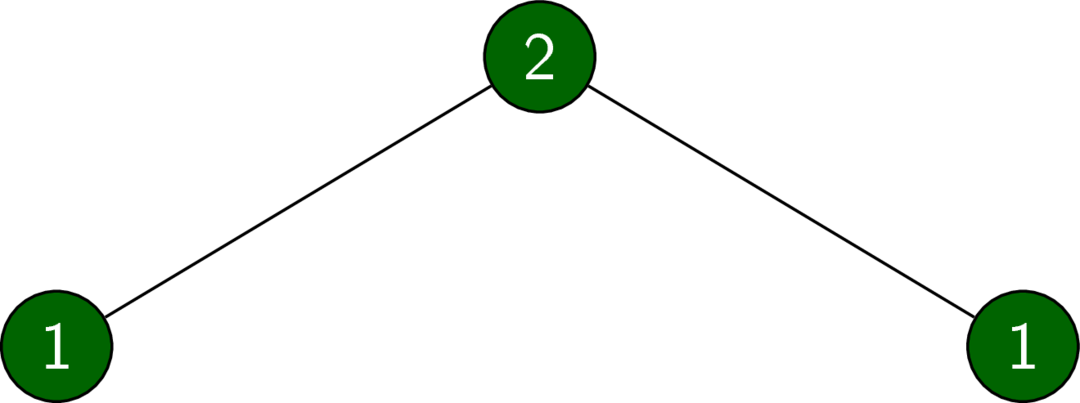A leftist tree with 3 nodes.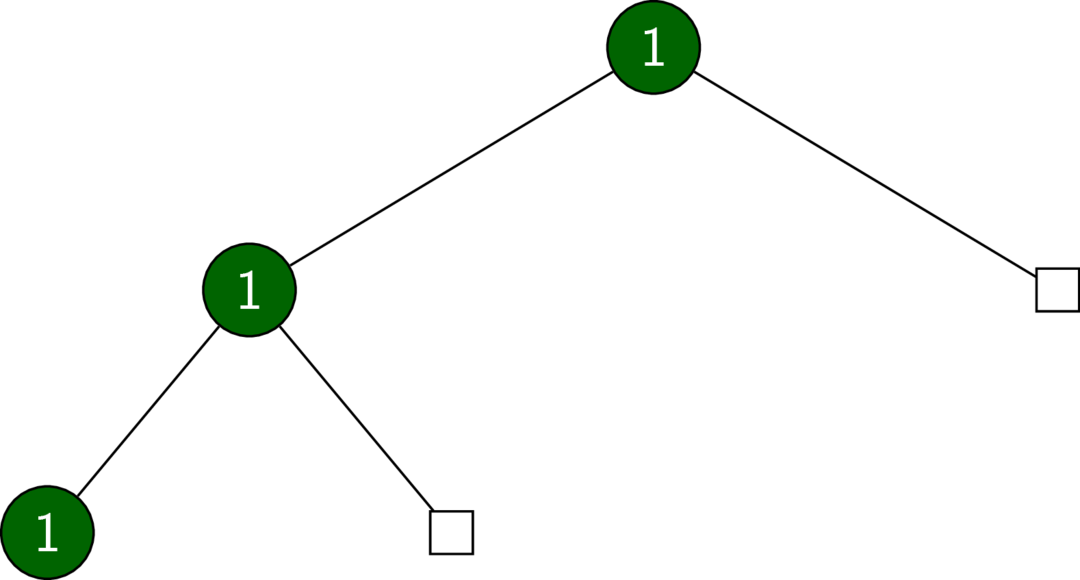A leftist tree with 3 nodes.

## Heaps

A tree is said to be heap-ordered if the key of any node is less than or equal to the key of any of its descendants. We capture this structure in the Heap typeclass.

class Heap h where
empty     :: Ord a => h a
isEmpty   :: Ord a => h a -> Bool
insert    :: Ord a =>   a -> h a -> h a
merge     :: Ord a => h a -> h a -> h a
findMin   :: Ord a => h a -> Maybe a     -- may be empty
deleteMin :: Ord a => h a -> Maybe (h a) -- may be empty

A leftist heap is a heap-ordered leftist tree. We can implement this as a binary tree with a heap instance.

## Exercise 3.1

Prove that the right spine of a leftist heap of size $$n$$ contains at most $$\left\lfloor \log (n+1) \right\rfloor$$ elements.

## Solution 3.1

We prove the stronger result that a leftist tree of rank $$r$$ is complete up to depth $$r-1$$. The solution then follows from the fact that a tree complete up to depth $$r-1$$ has at least $$2^r - 1$$ nodes.

The proof proceeds by induction on the number of nodes.

The statement is true for the empty tree.

Let $$T$$ be a leftist tree of rank $$r$$ with $$n$$ nodes. Then each child is a leftist tree with fewer nodes, so we may apply the induction hypothesis to each child. The right child has rank $$r-1$$ and, by the leftist property of $$T$$, the left child has rank at least $$r-1$$. By the induction hypothesis, each child is complete up to depth $$r-2$$. Therefore, $$T$$ is complete up to depth $$r-1$$. □

## Exercise 3.2

Define insert directly rather than via a call to merge.

See source.

## Exercise 3.3

Implement a function fromList of type

fromList :: Ord a => [a] -> LeftistHeap a

that produces a leftist heap from an unordered list of elements in $$\mathcal O (n)$$ time by merging in pairs.

## Solution 3.3

See source.

Note that we only use the Heap API, so we are guaranteed both the heap invariants and the leftist invariants. We must only check that the implementation is in fact linear.

Let’s look at the first few cases in detail.

• The first call to mergePairs calls merge a total of $$n/2$$ times on two heaps of size $$2^0$$. This has a cost of $$\frac{n}{2} \log 2 = \frac{n}{2}$$.

• The second call to mergePairs calls merge a total of $$n/4$$ times on heaps of size $$2^1$$. This has a cost of $$\frac{n}{4} \log 4 = \frac{n}{4} \cdot 2$$.

• The third call to mergePairs calls merge a total of $$n/8$$ times on heaps of size $$2^2$$. This has a cost of $$\frac{n}{8} \log 8 = \frac{n}{8} \cdot 3$$.

Indeed, the $$k$$th all to mergePairs calls merge a total of $$\frac{n}{2^k}$$ times on two heaps of size $$2^{k-1}$$. From this we see that the total cost is of order

$\sum_{k=1}^{\log n} \frac{n}{2^k} k = n \sum_{k=1}^{\log n} \frac{k}{2^k} .$

Since

$\frac{2i+1}{2^{2i+1}} + \frac{2i+2}{2^{2i+2}} \le \frac{1}{2^i} ,$

we can pair the terms in the sum to get a total cost of order

$n \sum_{k=0}^{\log n} \frac{1}{2^k} \le n \sum_{k=0}^{\infty} \frac{1}{2^k} \le 2n .$

## Exercise 3.4

A binary tree is said to satisfy the weight-biased leftist property if the size (= number of nodes) of any node’s left child is at least as large as that of its right child.

1. Prove that the right spine of a weight-biased leftist heap contains at most $$\left\lfloor \log (n+1) \right\rfloor$$ elements.
2. Modify the implementation in figure 3.2 to obtain weight-biased leftist heaps.
3. Modify merge for weight-biased leftist heaps to operate in a single top-down pass.
4. What advantages would the top-down version of merge have in a lazy environment? In a concurrent environment?

## Solution 3.4

Item 1.

The proof is almost identical to that for leftist trees in Exercise 3.1.

The statement is true for the empty tree.

Let $$T$$ be a weight-biased leftist heap of rank $$r$$ with $$n$$ nodes. Then each child is a weight-biased leftist tree with fewer nodes. The right child has rank $$r-1$$ and by the induction hypothesis must have at least $$2^{r-1}-1$$ nodes. By the weight-biased property of $$T$$, the left child also has at least $$2^{r-1}-1$$ nodes. Therefore, $$T$$ has at least $$2^r-1$$ nodes. In other words, $$\log (n+1) \ge r$$. Since $$r$$ is an integer, $$\left\lfloor \log (n+1) \right\rfloor \ge r$$. □

Item 2.

The implementation of leftist heaps encompasses both the leftist and weight-biased variants.

Item 3.

See source.

Item 4.

In a lazy environment, the top-down version has the advantage that some queries can be made to the merged heap without calculating the entire heap. For example, weight (merge h1 h2) would run in constant time regardless of the sizes of h1 and h2.

## Binomial Heaps

We define the binomial tree of rank 0 to be the singleton node.The binomial tree of rank 0

The binomial tree of rank r is defined as the tree formed by adding the binomial tree of rank n-1 as a left child of itself.The binomial tree of rank 1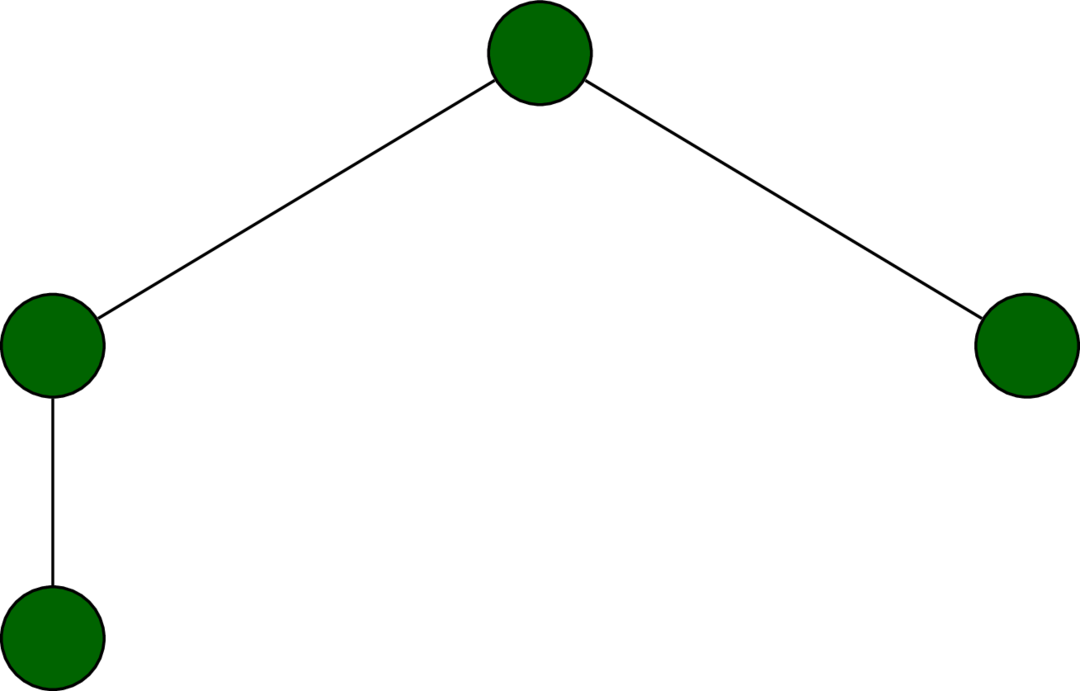The binomial tree of rank 2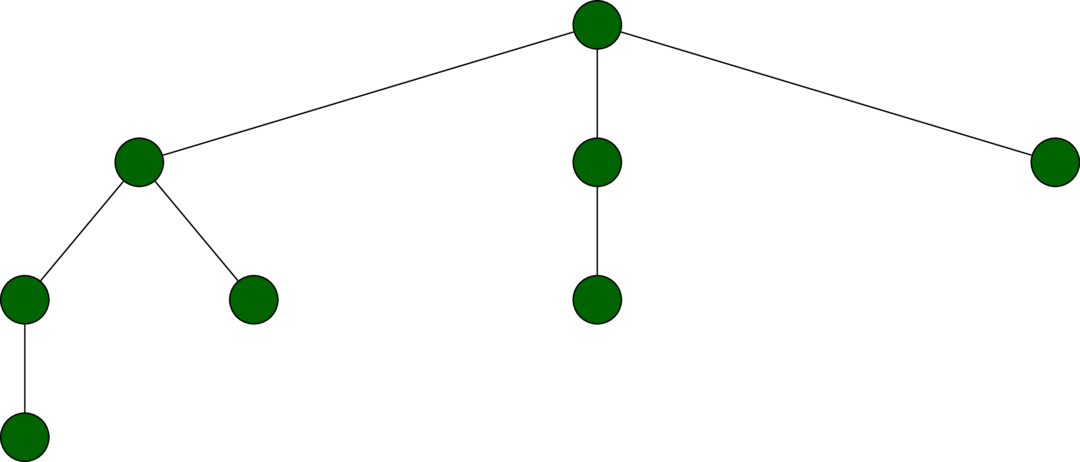The binomial tree of rank 3

Note that the binomial tree of rank r has exactly $$2^r$$ nodes. This follows from the fact that we double the number of nodes in the tree each time we increase the rank by 1.

There is an alternative definition of binomial trees. A binomial tree of rank $$r$$ is a node with $$r$$ children $$t_1, \dotsc, t_r$$, where $$t_i$$ is a binomial tree of rank $$r-i$$.

We represent a node in a binomial tree as a key with a list of children. The extra Int is to keep track of the rank.

data BinomialTree a = Node Int a [BinTree a]
deriving (Show, Eq)

We shall maintain two invariants:

1. Each list of children is in decreasing order of rank; and
2. The elements are stored in heap order.

A binomial heap is a forest of heap-ordered binomial trees, where no two trees in the forest have the same rank. Thus, the trees in a binomial heap of size n correspond to the 1s in the binomial representation of n. For example, a binomial heap of size 21 would have one tree of rank 4 (size $$2^4 = 16$$), one of rank 2 (size $$2^2=4$$), and one of rank 0 (size $$2^0=1$$), corresponding to 21’s binomial representation 10101. The binary representation of n contains $$\left\lfloor \log (n+1) \right\rfloor$$ digits, giving a bound for the number of trees in a binomial heap.

## Exercise 3.5

Define findMin directly rather than via a call to removeMinTree.

See source.

## Exercise 3.6

Given a binomial tree, the rank of the root determines the rank of the children. Reimplement binomial heaps without the redundant rank annotations.

See source.

## Exercise 3.7

Make a funtor ExplicitMin that creates a heap with a constant time findMin and logarithmic time deleteMin.

See source.

## Red-Black Trees

A red-black tree is a type of balanced binary search tree. The balance is achieved by painting the nodes either red or black whilst maintaining the following two invariants:

Red invariant
No red node has a red child
Black invariant
Every path from the root node to an empty node contains the same number of black nodes.

By convention, the empty node is defined to be black.The red-black tree of size 1The red-black tree of size 2The red-black tree of size 3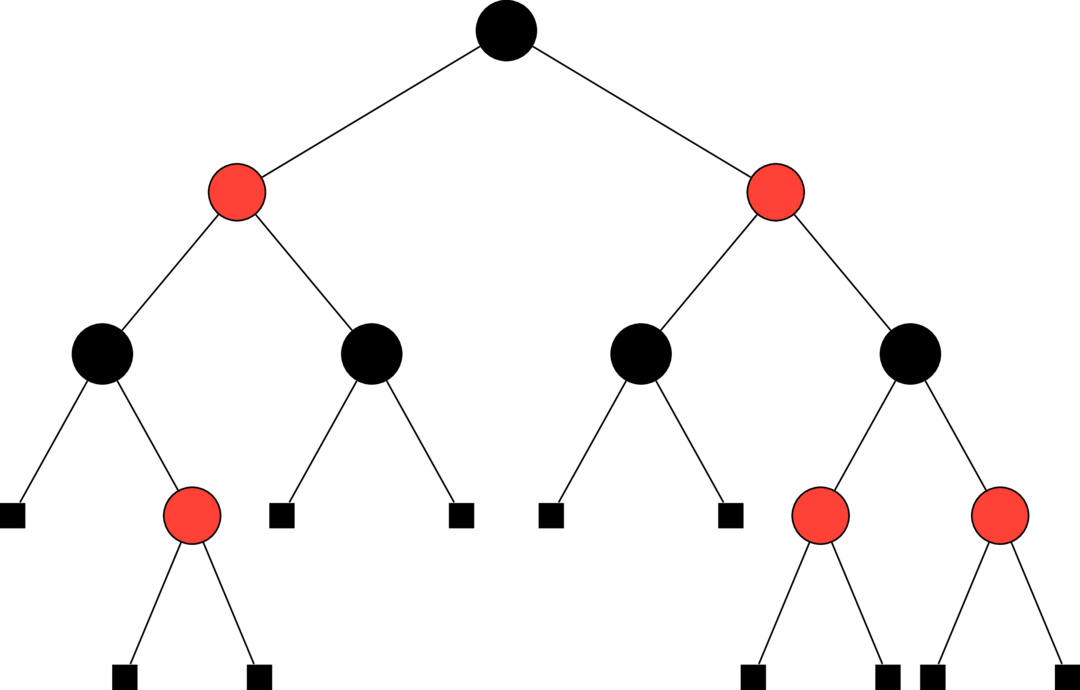A non-trivial red-black tree

Our data type is based on a BST with an extra colour field.

data Colour = R
| B
deriving (Show, Eq)

data RBTree a = E
| T Colour (RBTree a) a (RBTree a)
deriving (Eq)

## Exercise 3.8

Prove that the the maximum depth of a node in a red-black tree of size n is at most $$2\left\lfloor \log (n+1) \right\rfloor$$.

## Solution 3.8

We prove this by induction on the number of nodes.

The statement is true for empty trees.

Let $$T$$ be a red-black tree of depth $$d$$ with $$n$$ nodes. Suppose the trees rooted at its children have depths $$d_0$$ and $$d_1$$, with sizes $$n_0 \le n_1$$, respectively. In particular, the children are red-black trees with fewer than $$n$$ nodes. A consequence of the red-black invariant of $$T$$ is that $$d \le 2(d_0 + 1)$$, since $$d$$ is the length of the longest path and $$d_0$$ is at least the length of the shortest path. Applying the induction hypothesis to the children yields

\begin{align} d &\le 2(d_0 +1) \\ &\le 2(\log (n_0 + 1) + 1) \\ &= 2\log (2n_0 + 2) \\ &\le 2\log (n_0 + n_1 + 2) \\ &= 2\log (n + 1). \end{align}

## Exercise 3.9

Write a function fromOrdList of type

fromOrdList :: Ord a => [a] -> RedBlackTree a

that converts a sorted list with no duplicates into a red-black tree in $$\mathcal O (n)$$ time.

## Solution 3.9

See source.

We must prove both that this implementation has linear complexity and that the resulting tree is red-black invariant.

Proposition
The function fromOrdList has linear complexity.

The helper go makes at most two recursive calls to a list half the size of the original. More precisely,

$T (2k+1) = 2T (k) + \mathcal O (1)$

and

$T (2k) = T(k) + T (k-1) + \mathcal O (1).$

These are solved by $$T (n) = \mathcal O (n)$$.

For the invariants, note that the shape and colouring of the tree doesn’t depend on the particular elements of the list. With this in mind, we will prove a couple of lemmas.

Lemma
The black depth (as measured by the algorithm) of fromOrdList xs is exactly $$\left\lfloor \log (n+1) - 1\right\rfloor$$, where xs is a list of length $$n$$.

Proof. We prove this by induction on the length of the list.

For length $$0$$, the black depth of the resulting empty tree is $$-1 = \log (0 + 1) - 1$$.

By the induction hypothesis, the black depth in the odd length case $$n=2k+1$$ is $$\left\lfloor \log (k+1) \right\rfloor$$. This is equal to

\begin{align} & \quad \left\lfloor \log (k+1) \right\rfloor +1 -1 \\ &= \left\lfloor \log (k + 1) + \log 2 \right\rfloor - 1 \\ &= \left\lfloor \log (2k + 1 + 1) \right\rfloor - 1 \\ &= \left\lfloor \log (n + 1)\right\rfloor - 1. \end{align}

In the even case $$n=2k$$, the black depth is $$\left\lfloor \log k \right\rfloor$$, again using the induction hypothesis. This simplifies to

\begin{align} & \quad \left\lfloor \log k \right\rfloor +1 -1 \\ &= \left\lfloor \log 2k \right\rfloor - 1 \\ &= \left\lfloor \log (2k+1) \right\rfloor -1 \\ &= \left\lfloor \log (n+1) \right\rfloor - 1. \end{align}

The second line follows from the fact that $$\left\lfloor \log a \right\rfloor < \left\lfloor \log (a+1) \right\rfloor$$ if and only if $$a = 2^i - 1$$ for some $$i \in \mathbb N_{\ge 1}$$.

Proposition
The tree fromOrdList xs is black invariant for any list xs.

Proof. When the two recursive calls in go (length) xs produce trees of differing black depth, their black depths may differ by at most one. This follows from the previous lemma. By painting the tree with the larger black depth red, we maintain the invariant that both black depths are equal. Thus, the tree fromOrdList xs is black invariant.

Lemma
The root of the tree fromOrdList xs has a red left child if and only if the length of xs is $$2^i - 2$$ for some $$i \in \mathbb N_{\ge 2}$$. In any other case, both children are black.

Proof. Let $$n$$ be the size of the list xs. The function go (length xs) xs makes two recursive calls to create children of sizes approximately $$n/2$$. We paint the root of the left child red when their black depths differ, which can only occur if $$n = 2k$$ is even. Note that the left child always has the greater size and, by the previous lemma, the greater black depth. In this case the black depth of the left is $$\left\lfloor \log (k+1) \right\rfloor - 1$$, and the black depth of the right is $$\left\lfloor \log k \right\rfloor - 1$$. These differ precisely when $$k = 2^i - 1$$ for some $$i \in \mathbb N_{\ge 1}$$; that is, when $$n = 2 (2^i - 1) = 2^{i+1} - 2$$.

Proposition
The tree fromOrdList xs is red invariant for any list xs.

Proof. From the previous lemma, fromOrdList xs has a red left child, then then length of xs is $$n = 2^{i+1} - 2$$. But then the size of the left child is $$n/2 = 2^i - 1$$, so the left child has no red children. Therefore, no red invariant violation is introduced.

## Exercise 3.10

Reduce redundancy in the balance function as follows:

1. Split balance into two functions, lbalance and rbalance, that test for colour violations in the left and right child, respectively.
2. Rewrite ins so that it never tests the colour of nodes not on the search path.

## Solution 3.10

See source for both parts.